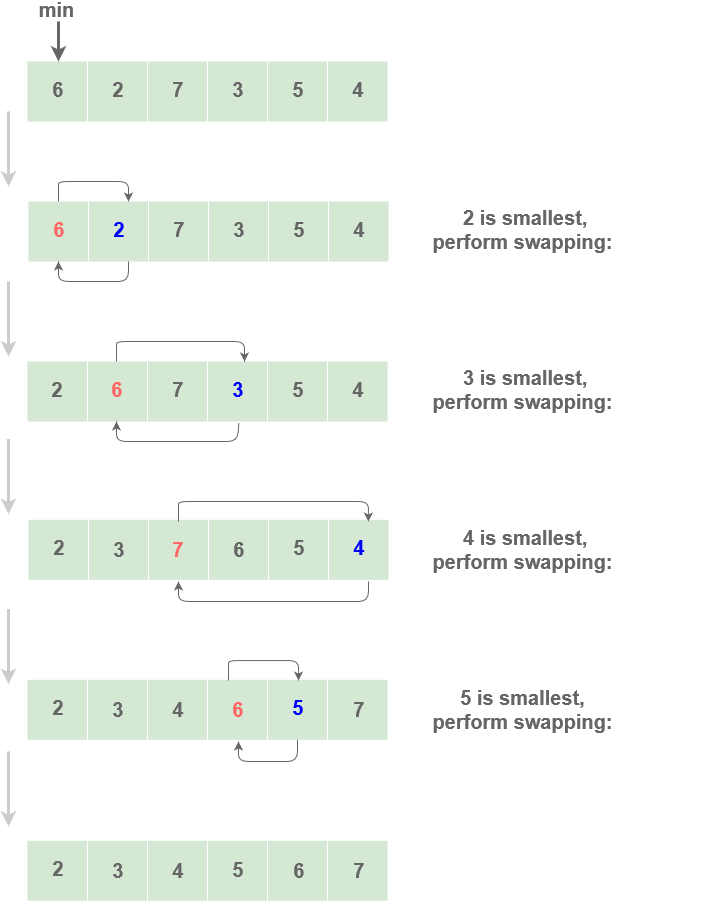# Selection Sort

## Selection Sort

Selection sort is an algorithm in which the smallest element from an unsorted list is selected in each iteration and placed at the beginning of the unsorted list.
Selection sort then selects the next-smallest element every time and swaps it into the correct place. This is why it is called selection sort

## Implementation

Steps

• Set the first element as `min`.
• Find the smallest element in the array and assign its index to `min`
• After each iteration, position the element at `min` to the front of the unsorted list.
• Start each iteration from the first unsorted element.
• Repeat the first three steps until list is sorted

Example :Code

``````void SelectionSort(int A[], int size)
{
for (int i = 0; i < size - 1; i++)
{
int min = i;//stores index of element with minimum value
for (int j = i + 1; j < i; j++)
{
if (A[j] < A[min])
min = j;
}
temp  = A[min];
A[min]= A[j];
A[j]  = temp;
}
}
``````

### Time Complexity : `O(N^2)`

Since there are 2 loops each running for ` O(n) `, the complexity is `O(N)*O(N)` = `O(N^2)`.

Scroll to Top

### Full Stack Integrated Bootcamp Free Trial

• Please enter a number from 7000000000 to 9999999999.